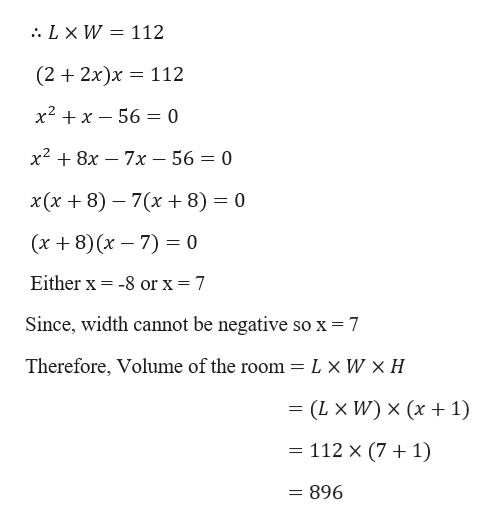# A boiler room has the shape of a rectangular prism. The area of the floor of the room is 112 square feet. The length of the room is 2 feet more than twice the width. The height is 1 foot greater than the width.What is the volume of the room in cubic feet? Do not include units in your answer.

Question
41 views

A boiler room has the shape of a rectangular prism. The area of the floor of the room is 112 square feet. The length of the room is 2 feet more than twice the width. The height is 1 foot greater than the width.

What is the volume of the room in cubic feet? Do not include units in your answer.

check_circle

Step 1

Given,

A boiler room has the shape of a rectangular prism. The area of the floor of the room is 112 square feet. The length of the room is 2 feet more than twice the width. The height is 1 foot greater than the width.

Let width of the room be x feet then

Length of the room will be 2 + 2x feet and

Height of the room will be x + 1 feet

Step 2

Now,

...help_outlineImage Transcriptionclose. LX W = 112 (2 2x)x 112 х2 +х — 56 — 0 х2 + 8х — 7х — 56 3 0 x (х + 8) — 7(х + 8) 3D 0 (x 8) (x 7) 0 Either x -8 or x = 7 Since, width cannot be negative so x = 7 Therefore, Volume of the room= Lx W x H = (L X W) x (x 1) 112 x (7 1) = 896 fullscreen

### Want to see the full answer?

See Solution

#### Want to see this answer and more?

Solutions are written by subject experts who are available 24/7. Questions are typically answered within 1 hour.*

See Solution
*Response times may vary by subject and question.
Tagged in

### Other